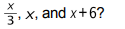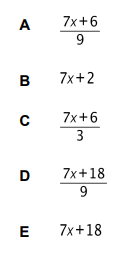# IMAT 2017 Q55 [Mean]

Which one of the following is an expression for the mean ofThe mean of these functions can be calculated by definition of an average number: A = \frac{1}{n}\sum_{i=1}^{n}a_i = \frac{a_1 + a_2 + \ldots + a_n}{n}

So in our case: A = \frac{(\frac{x}{3})+(x)+(x+6)}{3}=\frac{\frac{x + 3x + 3(x+6)}{3}}{3} = \frac{x + 3x + 3(x+6)}{9} = \frac{4x + 3x+18}{9} = \frac{7x+18}{9}.

So the correct answer is (D)